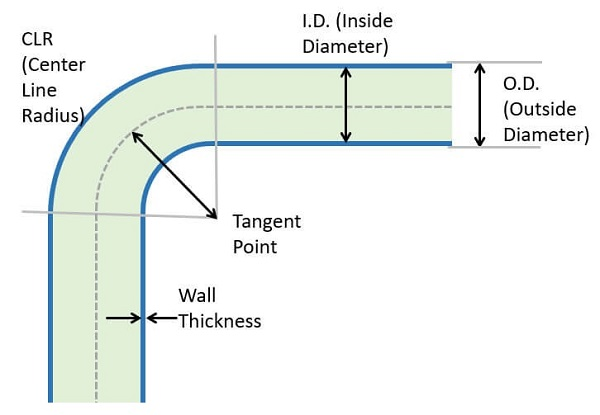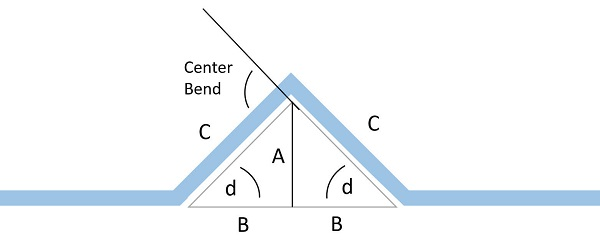Chapman Electric Supply, Inc. is now  part of Eckart Supply!  learn more>>

# Formulas for Calculating Conduit & Pipe Bends

## Calculations & Formulas

Using just a few mathematical formulas allows you to properly calculate a bend of nearly any angle. An inexpensive scientific calculator and an angle finder are the only additional tools required.

When calculating bend allowances to determine the cut length of HDPE conduit or PVC pipe, one must calculate from the center line radius (CLR) of the finished, bent pipe. This radius will vary depending on the outside diameter of the tube, the wall thickness, and the angle at which the tube is to be bent.

## Elements of a Bend

It is important to understand the different elements of a bend in order to make accurate calculations.Calculating Wall Thickness

ISO 161-1 uses the following formula to calculate the wall thickness of pipe:

σs=PN.(da-s/20.s)=PN.S

σs = hoop stress (N/mm2) | PN = normal pressure (bar) | da = external pipe diameter (mm)

s = wall thickness (mm) | S = pipe serial (-)

Calculating Standard Dimension Ratio

Using the same variables as above, the standard dimension ratio (SDR) of a pipe can be calculated thusly:

SDR = da/s

 HDPE Pipe SDR Minimum Long-TermCold Bending Radius 9 or less 20x pipe OD 11, 13.5 25x pipe OD 15.5, 17, 21 27x pipe OD 26 34x pipe OD 32.5 42x pipe OD 41 52x pipe OD With fitting or flangepresent in bend 100x pipe OD

Calculating CLR (Center Line Radius) for Bend Angle

After you’ve selected the appropriate die for bending your pipe, based on the pipe’s outside diameter and wall thickness, you should be able to find the radius of the bend.

A simple way to determine the center line radius of a bend of a specific angle is calculate a full circle, then divide that number by 360 to find the measurement of one degree. Then, use this formula:

π(2r) or πD

π (pi) = 3.1416

For example, if your die creates a 2.2” radius, and you need to create a 35° bend, your calculations would look something like this:

to calculate one degree of bend

3.1416(2x2.2) = 13.823/360 = 0.0384

to calculate CLR of 35° bend

0.0384 x 35 = 1.344”

Offset Bend CalculationMost bends other than 90° can be calculated using the geometry of a triangle. The black line represents an offset bend in a tube; the red triangle represents the triangular geometry this offset creates.

The lengths/sides of the triangle are labeled “a,” “b,” and “c”. The “d” represents the angle at which the pipe is bent. No matter how the tube is bent in this configuration (or how the triangle is oriented), one of the angles of the triangle will be 90°; the other angle will depend on the first angle (d), and can be calculated as (90 – d).

The relatively simple math formulas of sine, cosine, and tangent can be used to determine the angles of the triangle, and, therefore, the necessary angles of your pipe bend(s). Most scientific calculators (and even the calculators built into smart phones) have these functions.

Sine Calculation

Sine(d) = A/C

A = sine(d) x C

C = A/sine(d)

Cosine Calculation

Cos(d) = B/C

B = cos(d) x C

C = B/cos(d)

Tangent Calculation

Tan(d) = A/B

A = tan(d) x B

B = A/tan(d)

View information about bending conduit using a bender and the deducts and multipliers charts.

### Other Articles You Might Enjoy:

Choosing the Right Pipe for Underground Utility Applications

HDPE Pipe Welding & Joining